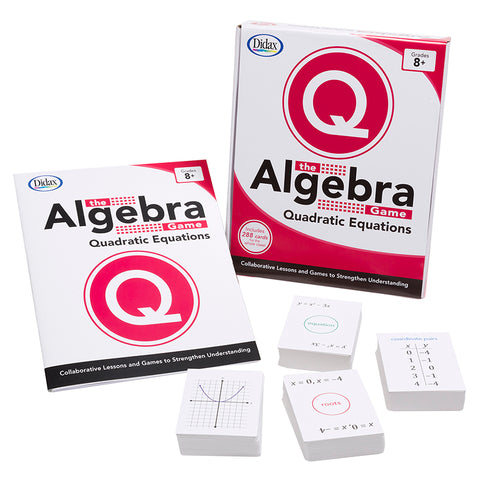## ALGEBRA GM QUADRATIC EQUATION BASIC

• 4999

The Algebra Game: Quadratic Equations Basic features an improved design and updated teaching instructions aligned to the current math standards.

Set includes a comprehensive teacher's guide featuring activities and games using the cards, discussion questions, and reproducible activities. Basic Guide includes the lessons that can also be used with Quadratic Equations Advanced set. Up to 12 students can work collaboratively to form a multitude of algebraic equations in each area of study.

In Quadratic Equations Basic students will discover the quadratic equation as they match the Basic cards with decks featuring factor, root, coordinate pair and graphs. Supplement with the Advanced set to match up cards featuring vertex, axis of symmetry, discriminant, and the quadratic formula.

Four decks (288 cards) plus 80-page guide.